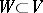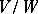# Representation theory

(diff) ← Older revision | Latest revision (diff) | Newer revision → (diff)

A theory studying homomorphisms of semi-groups (in particular, groups), algebras or other algebraic systems into corresponding endomorphism systems of suitable structures. Most often one considers linear representations, i.e. homomorphisms of semi-groups, groups, associative algebras, or Lie algebras into a semi-group, a group, an algebra, or a Lie algebra of linear transformations of a vector space. Such representations are also called linear representations in the space, andis called the representation space (or space of the representation). Frequently, representation theory means the theory of linear representations. Ifis finite-dimensional, then its dimension is called the dimension or degree of the representation, and the representation itself is called finite-dimensional. Thus, one distinguishes between finite-dimensional and infinite-dimensional representations. A representation is called faithful if it is injective (cf. Injection).

The study of linear representations of semi-groups, groups and Lie algebras also leads to the study of linear representations of associative algebras (cf. Representation of an associative algebra). More precisely, the linear representations of semi-groups (of groups) (cf. Representation of a group; Representation of a semi-group) in a spaceover a fieldare in a natural one-to-one correspondence with the representations of the corresponding semi-group (group) algebra overin. The representations of a Lie algebraovercorrespond bijectively to the linear representations of its universal enveloping algebra.

Specifying a linear representationof an associative algebrain a spaceis equivalent to specifying onan-module structure; thenis called the module of the representation. When considering representations of a groupor Lie algebra, one also speaks of-modules or-modules (cf. Module). Homomorphisms of modules of representations are called intertwining operators (cf. Intertwining operator). Isomorphic modules correspond to equivalent representations. A submodule of a moduleof a representationis a subspacethat is invariant with respect to; the representation induced inis called a subrepresentation and the representation induced in the quotient moduleis called a quotient representation of. Direct sums of modules correspond to direct sums of representations, indecomposable modules to indecomposable representations, simple modules to irreducible representations, and semi-simple modules to completely-reducible representations. The tensor product of linear representations, as well as the exterior and symmetric powers of a representation, also yield linear representations (cf. Tensor product of representations).

Next to abstract (or algebraic) representation theory there is a representation theory of topological objects, e.g., topological groups or Banach algebras (cf. Continuous representation; Representation of a topological group).